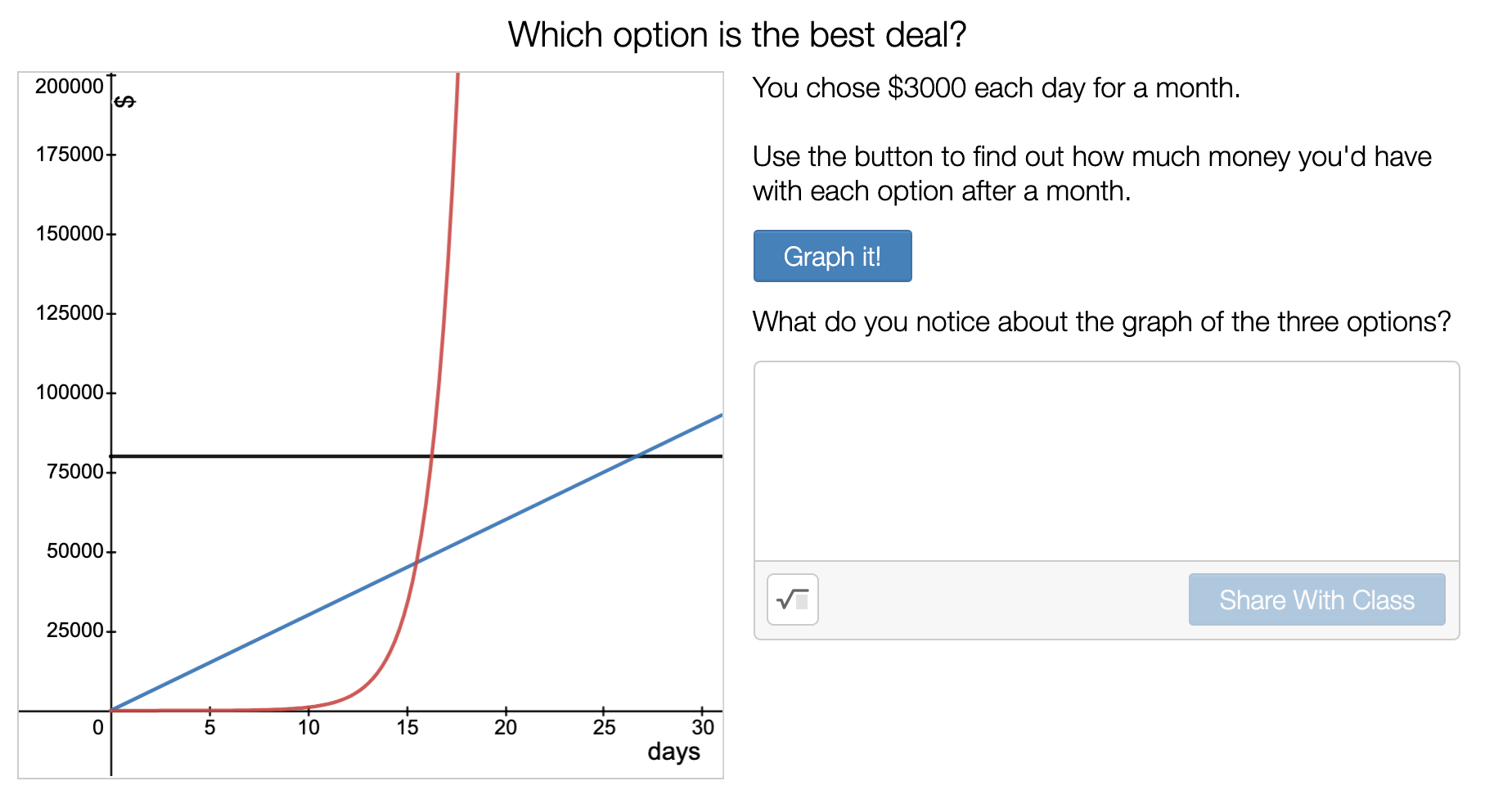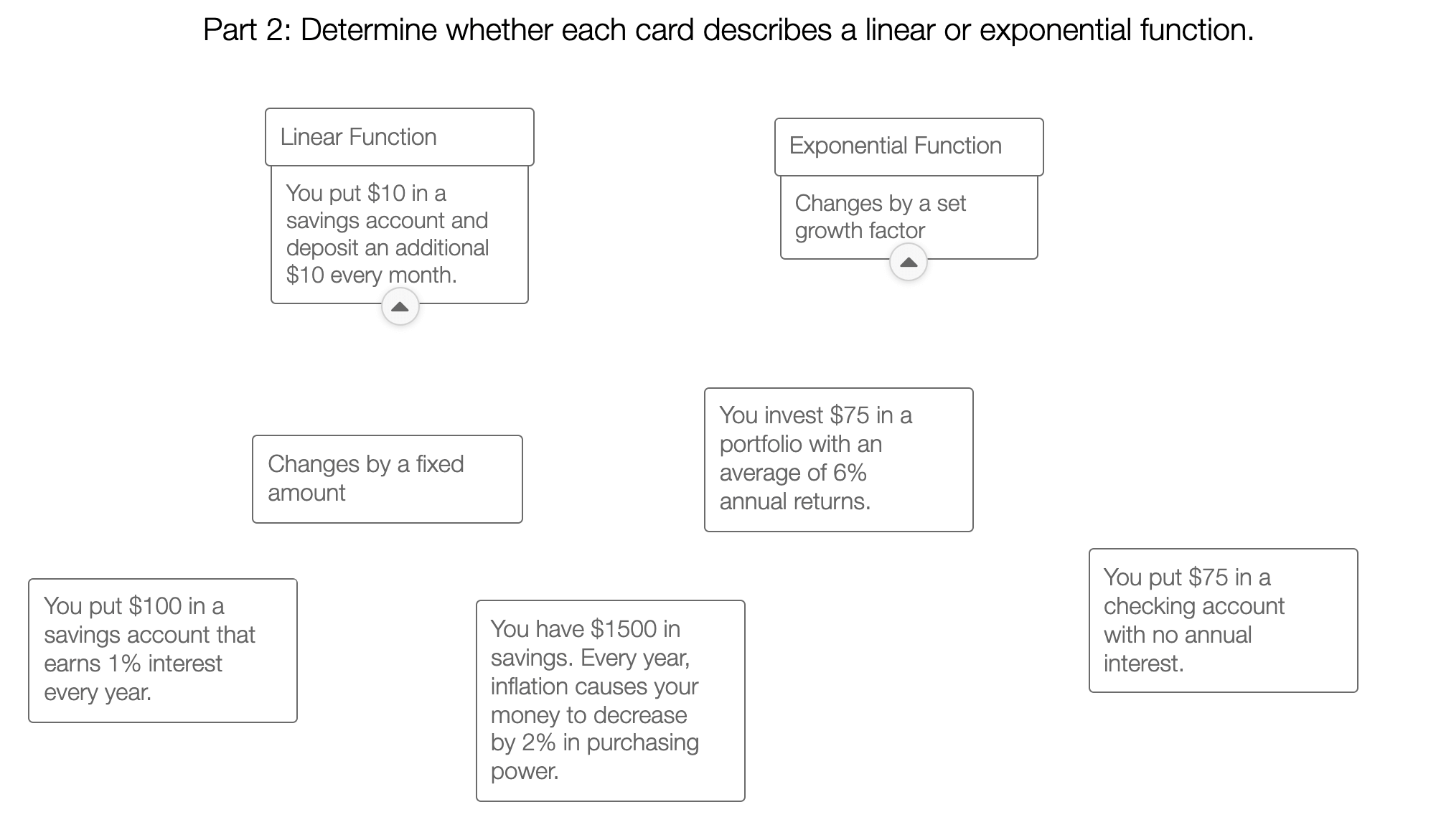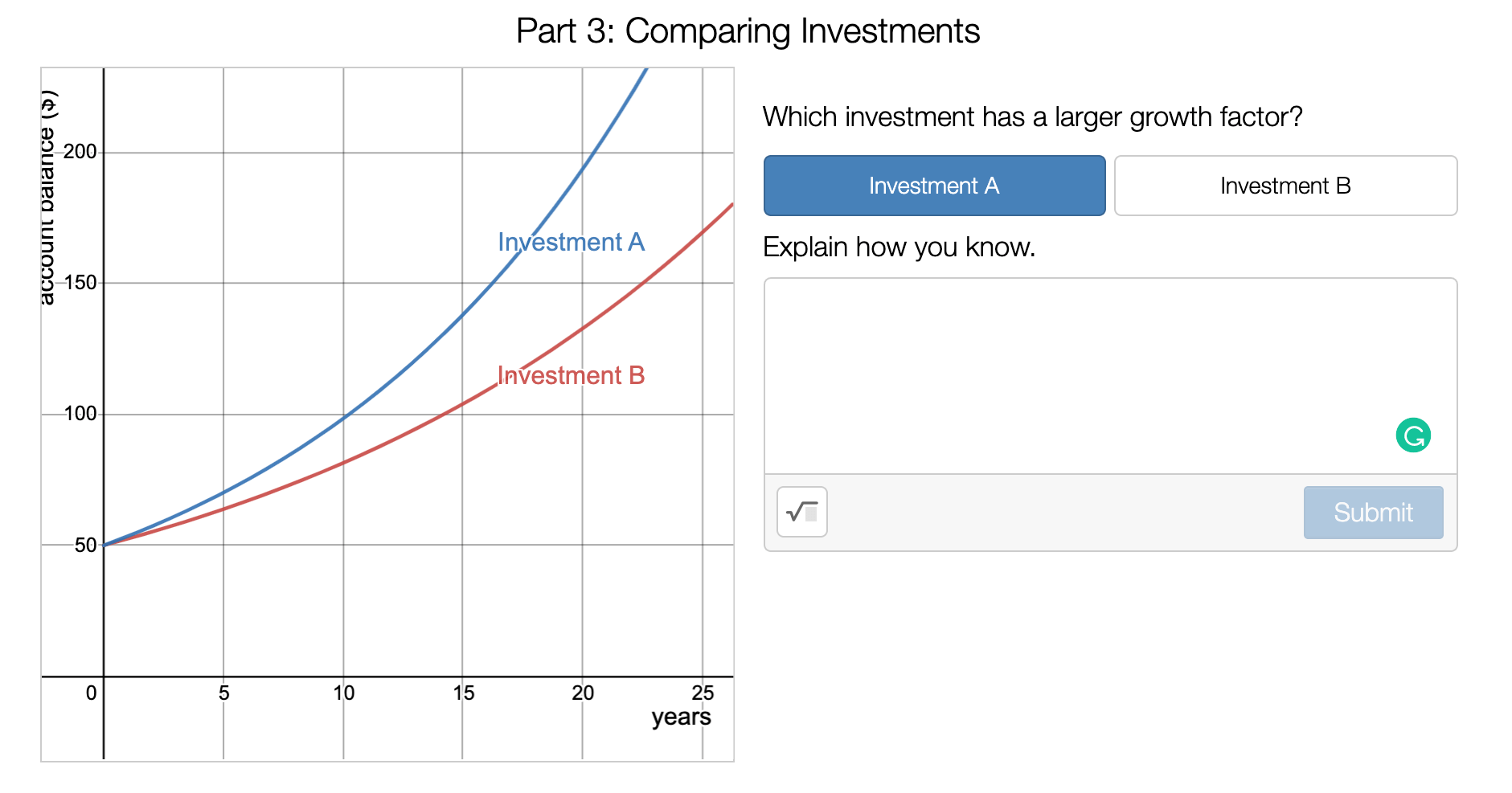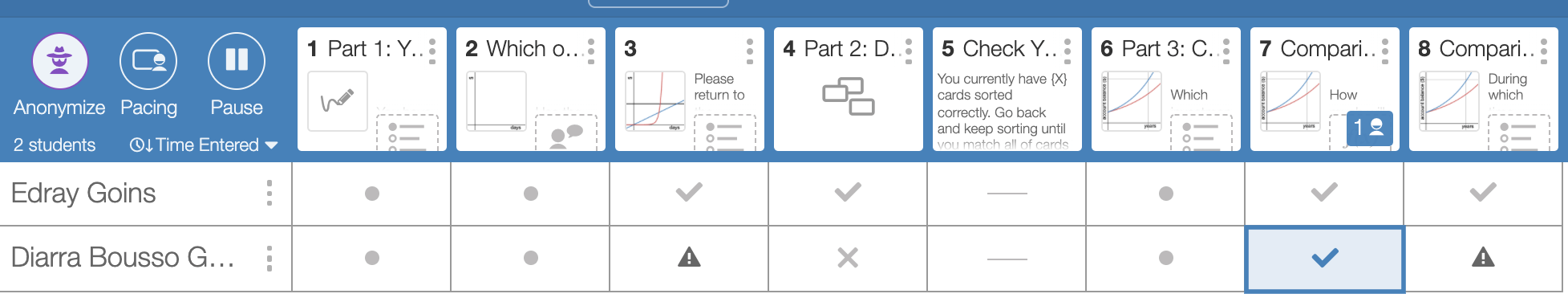Jan 09, 2022
Math

### Math Monday: Linear vs Exponential Growth in Investments

This Math Monday, we’re exploring a twist on the classic penny doubling problem to teach exponential functions.

In this Desmos activity, students compare three hypothetical ways they could collect a lottery prize: \$80,000 today, \$3000 per day for a month, or \$1 that doubles each day for a month. They also practice identifying linear vs exponential growth situations and comparing two exponential functions with different growth rates.

This activity corresponds to the Level 1 Application Problems for the Financial Algebra Lesson 5.2, where students are introduced to exponential growth patterns for the first time.

Part I: The Lottery

After choosing their lottery prize, students reveal the graph comparing the three options. The animated reveal of the graph highlights how quickly the doubling option starts to grow after 10 days.Next, students answer comprehension questions about the graph: when was the \$80,000 payment the best deal? Which function grows the fastest between days 10 and 20?

Part II: Linear or Exponential?

Students classify real-world checking and investing scenarios as either exponential or linear functions. They review growth rate and growth factor.Part III: Comparing Investment’s Growth Factors

Students are given the graph of two investments with the same initial value and different growth rates. They identify which investment had the larger growth factor (rate of return). Then, students answer four self-checking questions about the investments’ value and growth.Teacher Dashboard

As always, you can monitor student progress using the teacher dashboard. Much of this activity is self-checking, so you will also see a warning sign if students have attempted the same problem more than three times.Where to Find NGPF’s Desmos Activities

If you’re using our Financial Algebra curriculum, you’ll find Desmos activities built directly into the Lesson Guides and Application Answer Keys.

To look through NGPF’s library of Desmos activities, you can check out our Financial Algebra Collection.

Want More?

Want a great video to introduce your students to the stock market? Check out FinCap Friday: The Raging Bull!